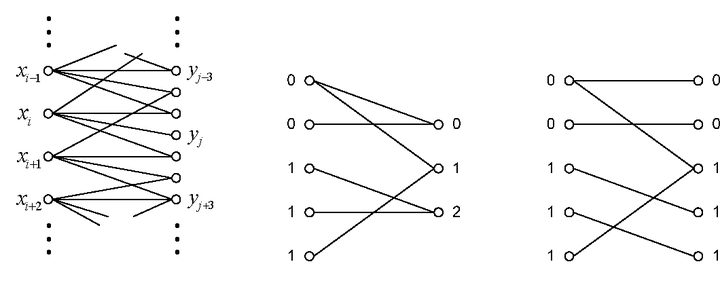# Analysis on Rate-distortion Performance of Compressive Sensing for Binary Sparse Source### Abstract

This paper proposes to use a bipartite graph to represent compressive sensing (CS). The evolution of nodes and edges in the bipartite graph, which is equivalent to the decoding process of compressive sensing, is characterized by a set of differential equations. One of main contributions in this paper is that we derive the close-form formulation of the evolution in statistics, which enable us to more accurately analyze the performance of compressive sensing. Based on the formulation, the distortion of random sampling and the rate needed to code measurements are analyzed briefly. Finally, numerical experiments verify our formulation of the evolution and the rate-distortion curves of compressive sensing are drawn to be compared with entropy coding.

Type
Publication
Data Compression Conference